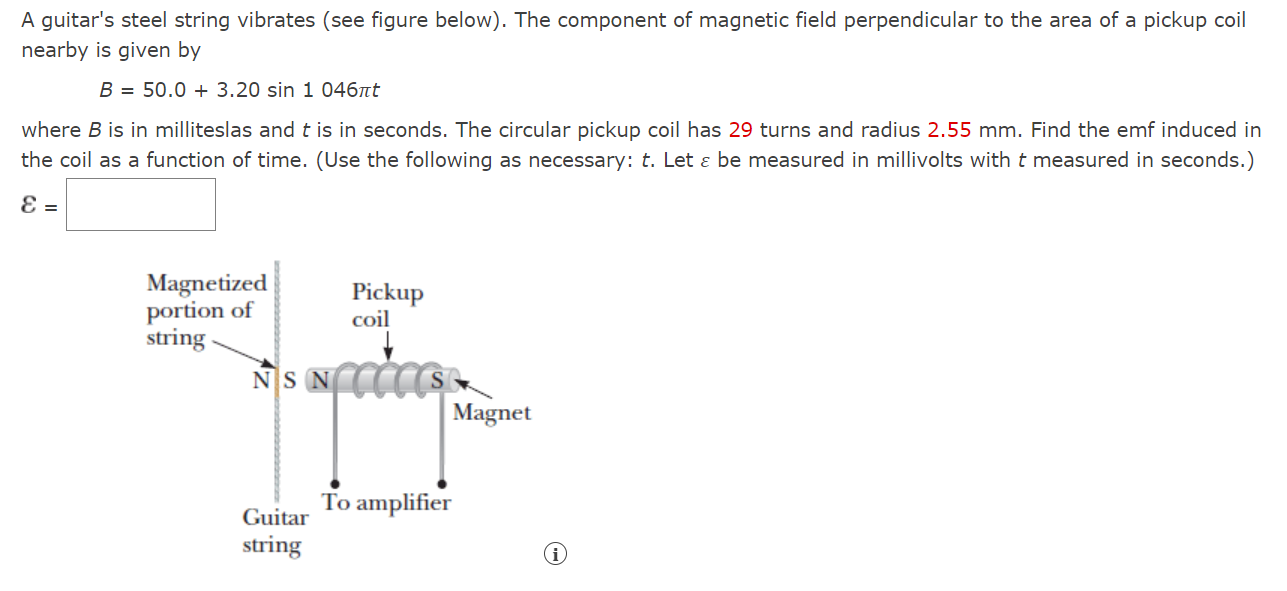Home / Expert Answers / Physics / a-guitar-39-s-steel-string-vibrates-see-figure-below-the-component-of-magnetic-field-perpendicular-t-pa420

# (Solved): A guitar's steel string vibrates (see figure below). The component of magnetic field perpendicular t ...

A guitar's steel string vibrates (see figure below). The component of magnetic field perpendicular to the area of a pickup coil nearby is given by

B = 50.0 + 3.20 sin 1 046????t

where B is in milliteslas and t is in seconds. The circular pickup coil has 29 turns and radius 2.55 mm. Find the emf induced in the coil as a function of time. (Use the following as necessary: t. Let ???? be measured in millivolts with t measured in seconds.)A guitar's steel string vibrates (see figure below). The component of magnetic field perpendicular to the area of a pickup coil nearby is given by where is in milliteslas and is in seconds. The circular pickup coil has 29 turns and radius . Find the emf induced in the coil as a function of time. (Use the following as necessary: . Let be measured in millivolts with measured in seconds.)

We have an Answer from Expert